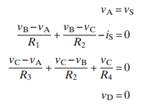### Create an Account

Already have account?

### Forgot Your Password ?

Home / Questions / The following are a set of node-voltage equations; draw the circuit they represent

# The following are a set of node-voltage equations; draw the circuit they represent

The following are a set of node-voltage equations; draw the circuit they represent.Jun 15 2020 View more View Less

#### Answer (Solved)Subscribe To Get Solution